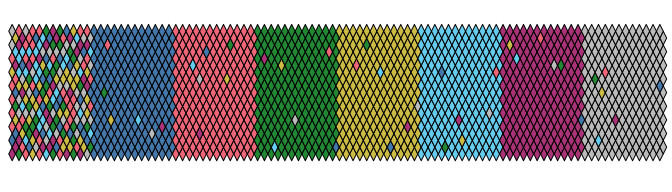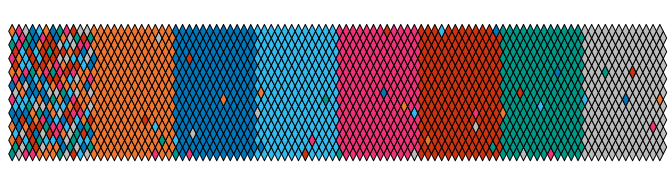# Paul Tol’s Colour Schemes

#### 2020-10-05

``library(khroma)``

# 1 Introduction

Tol (2018) offers carefully chosen schemes, ready for each type of data, with colours that are:

• Distinct for all people, including colour-blind readers.
• Distinct from black and white.
• Distinct on screen and paper.
• Matching well together.

All the scales presented in Paul Tol’s technical note (issue 3.1, 2018-09-23) are implemented here, for use with base R `graphics` or `ggplot2`.

# 2 Qualitative data

According to Paul Tol’s technical note, the bright, contrast, vibrant and muted colour schemes are colour-blind safe.

The light colour scheme is reasonably distinct for both normal or colour-blind vision and is intended to fill labelled cells.

The pale and dark schemes are not very distinct in either normal or colour-blind vision and should be used as a text background or to highlight a cell in a table.

The qualitative colour schemes must be used as given (no interpolation): colours are picked up to the maximum number of supported values. Refer to the original document for details about the recommended uses (see references).

Scheme Max. colours
bright 7
contrast 3
vibrant 7
muted 9
pale 6
dark 6
light 9

## 2.1bright

``````bright <- colour("bright")
plot_scheme(bright(7), colours = TRUE, names = TRUE, size = 0.9)``````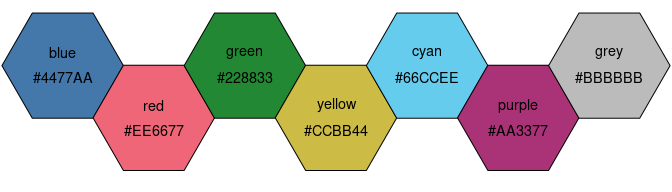## 2.2contrast

``````contrast <- colour("contrast")
plot_scheme(contrast(3), colours = TRUE, names = TRUE, size = 0.9)``````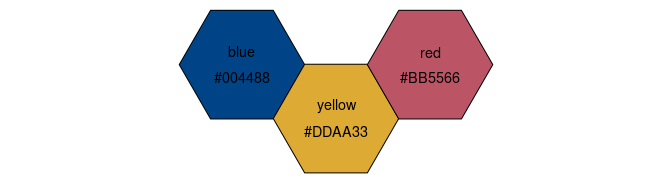## 2.3vibrant

``````vibrant <- colour("vibrant")
plot_scheme(vibrant(7), colours = TRUE, names = TRUE, size = 0.9)``````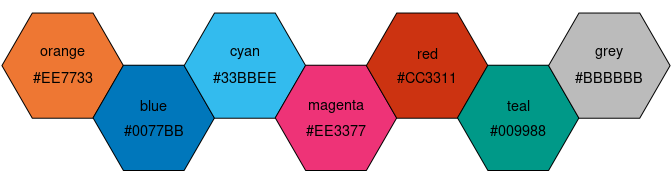## 2.4muted

``````muted <- colour("muted")
plot_scheme(muted(9), colours = TRUE, names = TRUE, size = 0.9)``````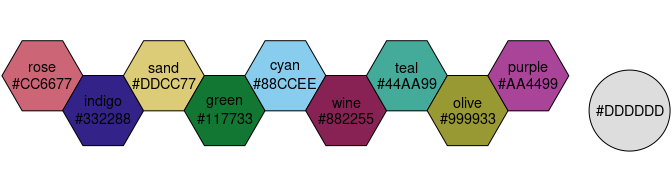## 2.5light

``````light <- colour("light")
plot_scheme(light(9), colours = TRUE, names = TRUE, size = 0.9)``````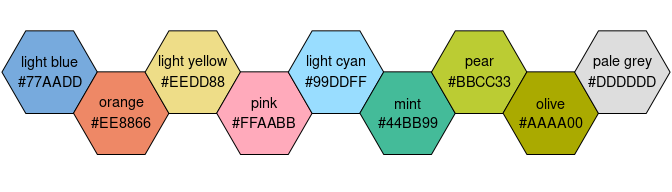## 2.6pale and dark

``````pale <- colour("pale")
plot_scheme(pale(6), colours = TRUE, names = TRUE, size = 0.9)``````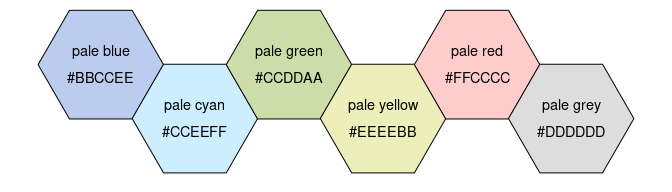``````
dark <- colour("dark")
plot_scheme(dark(6), colours = TRUE, names = TRUE, size = 0.9)``````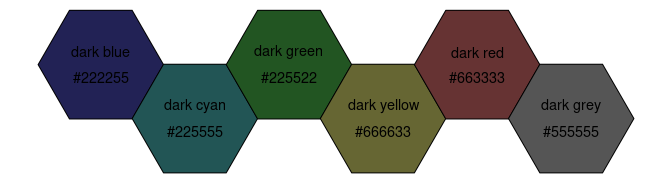# 3 Diverging data

If more colours than defined are needed from a given scheme, the colour coordinates are linearly interpolated to provide a continuous version of the scheme.

Scheme Num. of colours Bad data
sunset 11 #FFFFFF
BuRd 9 #FFEE99
PRGn 9 #FFEE99

## 3.1sunset

``````sunset <- colour("sunset")
plot_scheme(sunset(9), colours = TRUE, size = 0.9)``````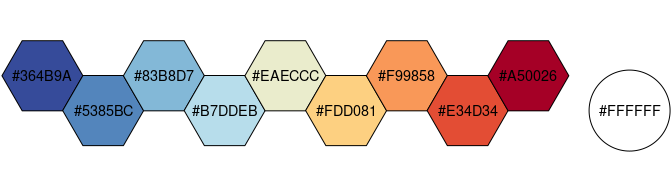## 3.2BuRd

``````BuRd <- colour("BuRd")
plot_scheme(BuRd(9), colours = TRUE, size = 0.9)``````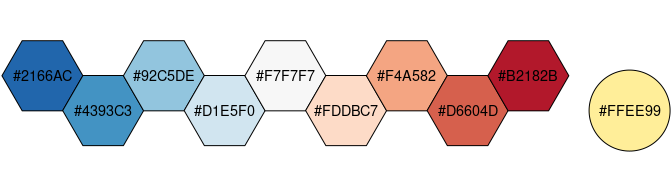## 3.3PRGn

``````PRGn <- colour("PRGn")
plot_scheme(PRGn(9), colours = TRUE, size = 0.9)``````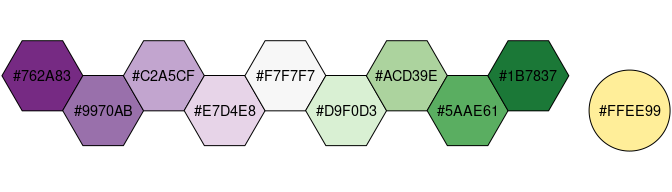# 4 Sequential data

If more colours than defined are needed from a given scheme, the colour coordinates are linearly interpolated to provide a continuous version of the scheme, with the exception of the discrete rainbow scheme (see below).

Scheme Num. of colours Bad data
YlOrBr 9 #888888
iridescent 23 #999999
discrete rainbow 23 #777777
smooth rainbow 34 #666666

## 4.1YlOrBr

``````YlOrBr <- colour("YlOrBr")
plot_scheme(YlOrBr(9), colours = TRUE, size = 0.9)``````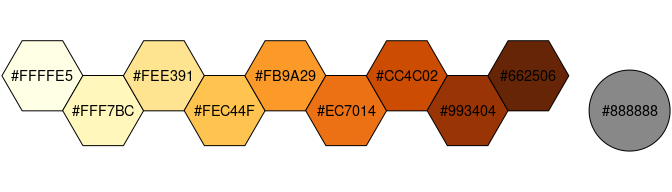## 4.2iridescent

``````iridescent <- colour("iridescent")
plot_scheme(iridescent(23), colours = TRUE, size = 0.5)``````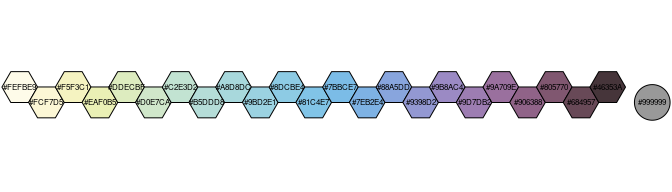## 4.3rainbow

As a general rule, ordered data should not be represented using a rainbow scheme. There are three main arguments against such use (Tol 2018):

• The spectral order of visible light carries no inherent magnitude message.
• Some bands of almost constant hue with sharp transitions between them, can be perceived as jumps in the data.
• Colour-blind people have difficulty distinguishing some colours of the rainbow.

If such use cannot be avoided, Paul Tol’s technical note provides two colour schemes that are reasonably clear in colour-blind vision. To remain colour-blind safe, these two schemes must comply with the following conditions:

discrete rainbow
This scheme must not be interpolated.
smooth rainbow
This scheme does not have to be used over the full range (Tol 2018 suggests starting at purple).
``````discrete_rainbow <- colour("discrete rainbow")
plot_scheme(discrete_rainbow(14), colours = TRUE, size = 0.7)``````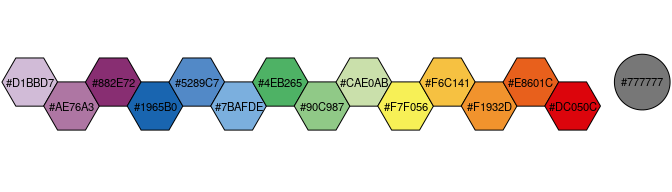When using the smooth rainbow scheme:

• Start off-white instead of purple if the lowest data value occurs often;
• End at red instead of brown the highest data value occurs often.
``````smooth_rainbow <- colour("smooth rainbow")

# Start at purple instead of off-white
plot(smooth_rainbow(256, range = c(0.25, 1)))````````````# End at red instead of brown
plot(smooth_rainbow(256, range = c(0, 0.9)))``````# 5 Diagnostic maps

## 5.1 Qualitative colour schemes